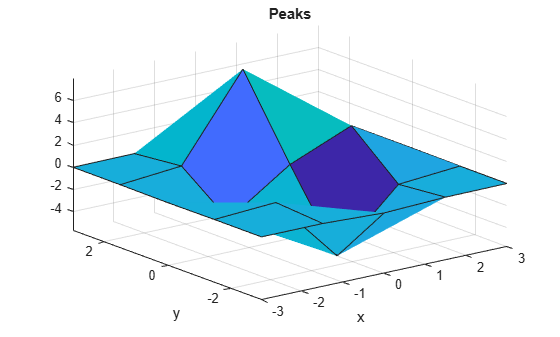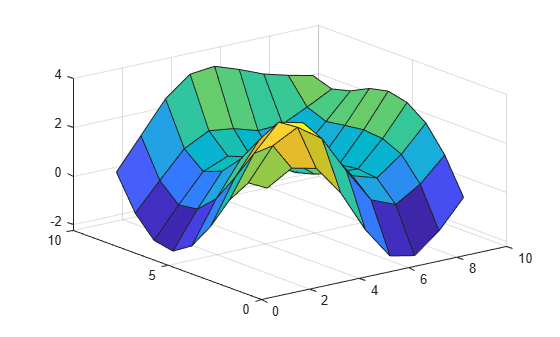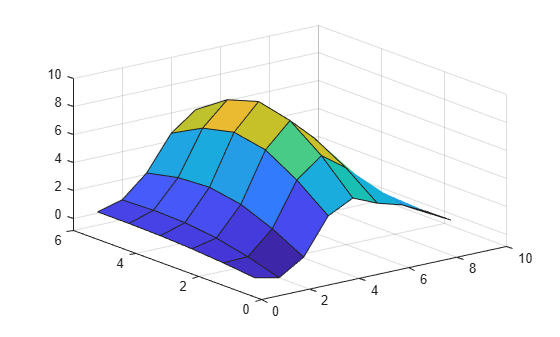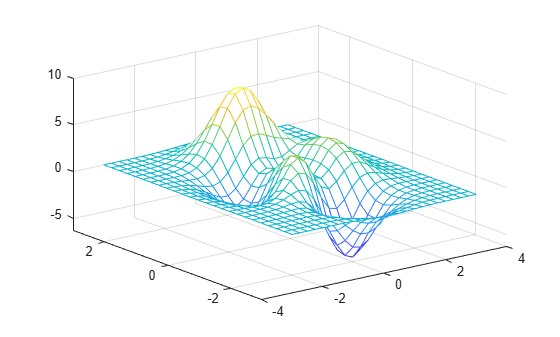Main Content

# peaks

Peaks function

## Syntax

``Z = peaks``
``Z = peaks(n)``
``Z = peaks(Xm,Ym)``
``[X,Y,Z] = peaks(___)``
``peaks(___)``

## Description

### Return Coordinates

````Z = peaks` returns the z-coordinates of the peaks function evaluated over a 49-by-49 grid.```

example

````Z = peaks(n)` returns the peaks function evaluated over an `n`-by-`n` grid. If you specify `n` as a vector of length k, MATLAB® evaluates the function over a k-by-k grid.```

example

````Z = peaks(Xm,Ym)` returns the peaks function evaluated at the points specified by `Xm` and `Ym`. The sizes of `Xm` and `Ym` must be the same or be compatible. For example, you can specify `Xm` and `Ym` as matrices in `meshgrid` format.```

example

````[X,Y,Z] = peaks(___)` returns the x-, y-, and z-coordinates of the peaks function.```

### Create Surface Plot

example

````peaks(___)` plots the peaks function as a three-dimensional surface plot. You can use any of the input argument combinations in the previous syntaxes. ```

## Examples

collapse all

Create a 5-by-5 matrix of peaks and display the surface.

```figure peaks(5)```
``` z = 3*(1-x).^2.*exp(-(x.^2) - (y+1).^2) ... - 10*(x/5 - x.^3 - y.^5).*exp(-x.^2-y.^2) ... - 1/3*exp(-(x+1).^2 - y.^2) ```Evaluate the peaks function over a 2-D grid that is defined by uniformly spaced values in the interval [-1,1].

```n = -1:0.25:1; Z = peaks(n)```
```Z = 9×9 1.8559 2.4537 2.2247 0.9965 -0.7239 -2.0151 -2.2222 -1.4251 -0.2729 1.7829 3.0862 3.6265 2.9423 1.3962 -0.0048 -0.4134 0.2037 1.1760 0.7969 2.4879 3.5507 3.3044 1.9975 0.7001 0.3896 1.1388 2.1978 -0.5659 1.2281 2.5614 2.6254 1.5850 0.5256 0.4756 1.4962 2.7556 -1.6523 0.0636 1.4796 1.7530 0.9810 0.1904 0.3744 1.5728 2.9369 -2.1491 -0.6469 0.6823 1.0566 0.5228 -0.0281 0.2795 1.4682 2.7624 -1.9968 -0.7599 0.3898 0.7963 0.4641 0.0898 0.3754 1.3442 2.3768 -1.1629 -0.0458 1.0400 1.5519 1.4226 1.1205 1.1713 1.6492 2.1708 0.2289 1.5069 2.7942 3.5855 3.6886 3.3404 2.9344 2.6622 2.4338 ```

Use the calculated values to create a three-dimensional surface plot.

`surf(Z)`Create 2-D grid coordinates with x-coordinates defined by the vector `x` and y-coordinates defined by the vector `y`.

```x = -2:0.5:2; y = 1:0.2:2; [X,Y] = meshgrid(x,y);```

Evaluate the peaks function over the 2-D grid.

`Z = peaks(X,Y)`
```Z = 6×9 -0.4808 -0.8639 0.2289 2.7942 3.6886 2.9344 2.4338 1.5813 0.5805 -0.2470 -0.1924 1.4275 4.5254 5.8902 4.6339 2.8647 1.3897 0.4382 -0.0730 0.3116 2.3394 5.8525 7.5679 5.9243 3.2006 1.2552 0.3350 0.0320 0.5860 2.7339 6.2839 8.1000 6.3260 3.2091 1.1050 0.2561 0.0764 0.6437 2.5958 5.7476 7.3967 5.7698 2.8374 0.9069 0.1901 0.0797 0.5539 2.0967 4.5569 5.8591 4.5675 2.2099 0.6771 0.1328 ```

Use the calculated values to create a three-dimensional surface plot.

`surf(Z)`Use the `peaks` function to create three matrices of the same size. Then, plot them as a mesh plot.

```[X,Y,Z] = peaks(25); mesh(X,Y,Z)```## Input Arguments

collapse all

Value representing a 2-D grid used to evaluate the function, specified as a scalar or vector.

• If `n` is a scalar, MATLAB creates the grid as ```[X,Y] = meshgrid(linspace(-3,3,n))```. The value of `n` must be an integer greater than 1.

• If `n` is a vector, MATLAB creates the grid as `[X,Y] = meshgrid(n)`. The elements of `n` must be double-precision numbers.

For example, `Z = peaks(5)` is the same as using this code.

```[X,Y] = meshgrid(linspace(-3,3,5)); Z = peaks(X,Y) ```

Example: `n = 5`

Example: `n = -2:0.1:2`

x-coordinates of points, specified as a scalar, vector, or matrix. The sizes of `Xm` and `Ym` must be the same or be compatible. You can use the `meshgrid` function to create `Xm` and `Ym`. See Compatible Array Sizes for Basic Operations for more information about compatible arrays.

Example: `Xm = [1 2 3; 1 2 3; 1 2 3]`

Example: `[Xm,Ym] = meshgrid(-5:0.5:5)`

Data Types: `single` | `double` | `int8` | `int16` | `int32` | `int64` | `uint8` | `uint16` | `uint32` | `uint64`

y-coordinates of points, specified as a scalar, vector, or matrix. The sizes of `Xm` and `Ym` must be the same or be compatible. You can use the `meshgrid` function to create `Xm` and `Ym`. See Compatible Array Sizes for Basic Operations for more information about compatible arrays.

Example: `Ym = [1 1 1; 2 2 2; 3 3 3]`

Example: `[Xm,Ym] = meshgrid(-5:0.5:5)`

Data Types: `single` | `double` | `int8` | `int16` | `int32` | `int64` | `uint8` | `uint16` | `uint32` | `uint64`

## Output Arguments

collapse all

x-coordinates of points, returned as a scalar, vector, or matrix. If `peaks` is called with the `Xm` and `Ym` input arguments, then `X` is equal to `Xm`. Otherwise, `X` is a matrix that specifies the x-coordinates over a grid used for evaluating the peaks function.

y-coordinates of points, returned as a scalar, vector, or matrix. If `peaks` is called with the `Xm` and `Ym` input arguments, then `Y` is equal to `Ym`. Otherwise, `Y` is a matrix that specifies the y-coordinates over a grid used for evaluating the peaks function.

z-coordinates of points, returned as a scalar, vector, or matrix.

## More About

collapse all

### Peaks Function

The peaks function is useful for demonstrating graphics functions, such as `contour`, `mesh`, `pcolor`, and `surf`. It is obtained by translating and scaling Gaussian distributions and is defined as

`$z=3{\left(1-x\right)}^{2}{e}^{-{x}^{2}-{\left(y+1\right)}^{2}}-10\left(\frac{x}{5}-{x}^{3}-{y}^{5}\right){e}^{-{x}^{2}-{y}^{2}}-\frac{1}{3}{e}^{-{\left(x+1\right)}^{2}-{y}^{2}}.$`

## See Also

Introduced before R2006a

Download ebook